International
Tables for
Crystallography
Volume B
Reciprocal space
Edited by U. Shmueli

International Tables for Crystallography (2006). Vol. B, ch. 5.1, pp. 547-548   | 1 | 2 |

## Section 5.1.7.2.2. Absorbing crystals

A. Authiera*

aLaboratoire de Minéralogie-Cristallographie, Université P. et M. Curie, 4 Place Jussieu, F-75252 Paris CEDEX 05, France
Correspondence e-mail: authier@lmcp.jussieu.fr

#### 5.1.7.2.2. Absorbing crystals

| top | pdf |

Reflected intensity. The intensity of the reflected wave for a thin absorbing crystal is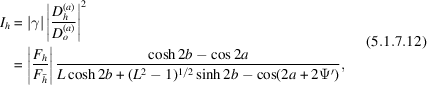where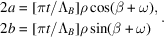L, ρ and ψ′ are defined in (5.1.7.5), β is defined in (5.1.3.7)and ω is the phase angle of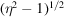.

Comparison with geometrical theory. When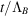decreases towards zero, expression (5.1.7.12)tends towards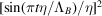; using (5.1.3.5)and (5.1.3.8), it can be shown that expression (5.1.7.12)can be written, in the non-absorbing symmetric case, as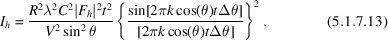where d is the lattice spacing and Δθ is the difference between the angle of incidence and the middle of the reflection domain. This expression is the classical expression given by geometrical theory [see, for instance, James (1950)].

### References

James, R. W. (1950). The optical principles of the diffraction of X-rays. London: G. Bell and Sons Ltd.Google Scholar